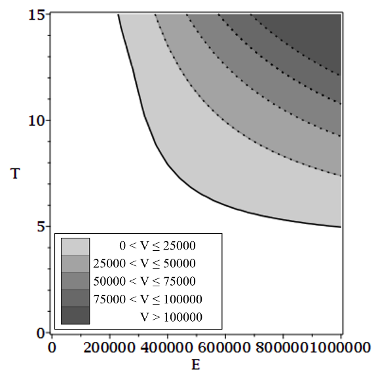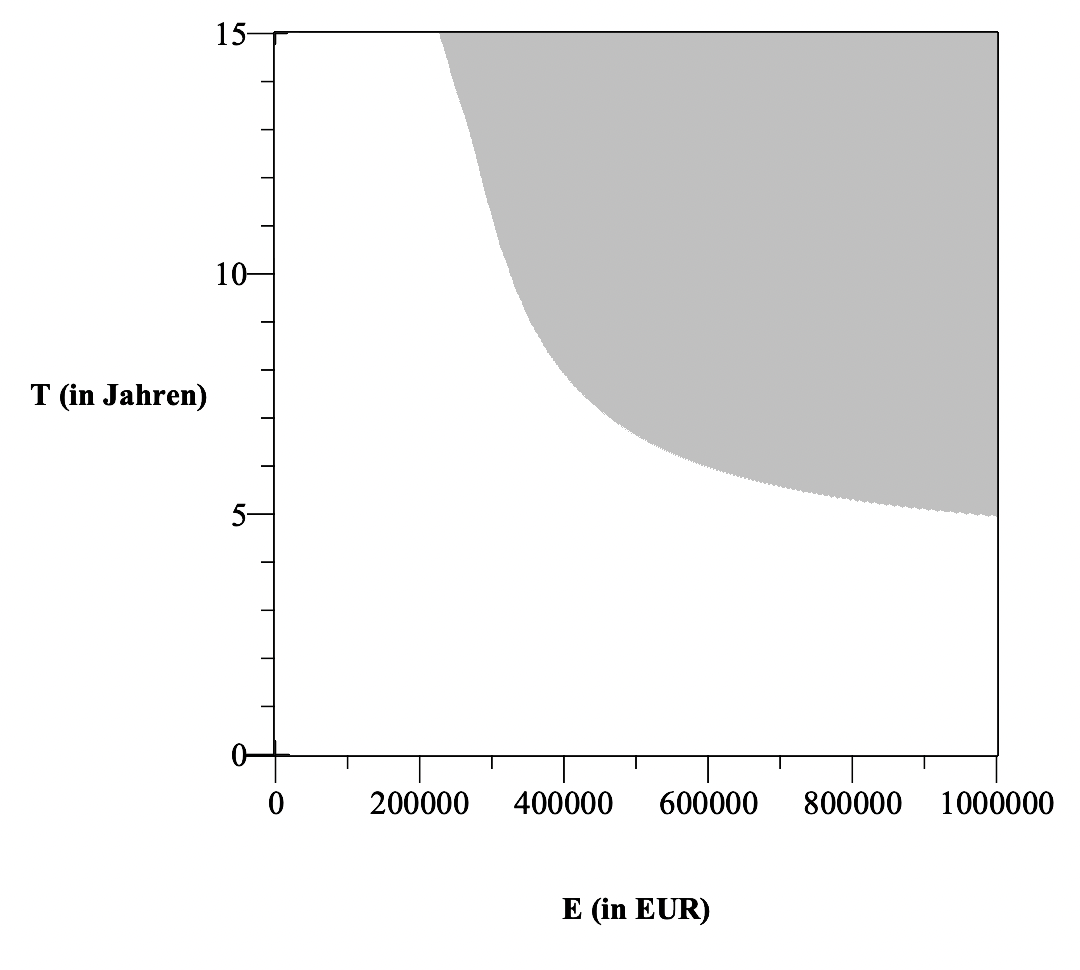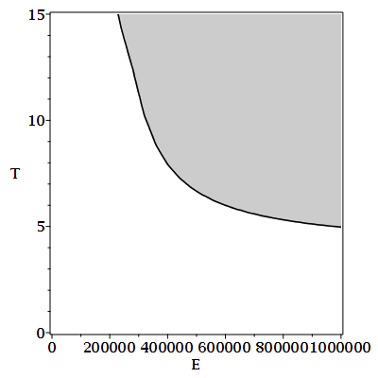## MaplePrimes Questions

Search Questions:

## How do I implement expandable connectors from Mode...

Hi there!

I'm working on implementing a custom Modelica Library in MapleSim 2021. I have Maple 2021 installed and my software is up to date. The library I have developed is in a single file (extension ".mo") which I developed on an IDE for Modelica i.e., I did not create the library using MapleSim. During the import into MapleSim, no errors appear in the system logs. All my components and models have been imported except for an "expandable connector". It appears that the problem is with the term "expandable".

Since this expandable connector does not appear among my library components, I attempted to create a custom component using the Modelica code editor in MapleSim. However, the file cannot be saved while I prefix the term "expandable" to "connector". The software allows me to save the file with the new code after dropping the "expandable" term.

I know that expandable connectors are used by Modelica. Here are the references I used during development:

https://mbe.modelica.university/components/architectures/expandable/

Working with Expandable Connectors - Claytex

However, there does not seem to be any information on expandable connectors in MapleSim. I'd appreciate it if any of you could throw some light on why I'm not able to import this component into MapleSim and fixes/suggestions on what I might be doing wrong. If any further information on my question is required, please do let me know.

## During evaluating function...

During evaluating psi0 (see eq, (7))why we need two values of f (i.e., f,0 and f,3)? I asked one of my seniors and according to him these values are arbitrary. Can anyone explain why we need two values and why f,0 and f,3?

## Plot the energy of a system of coupled oscillators...Hello. I will post 4 images of what I want to ask because I think it is a little difficult for me to describe it. I want to plot the energy transfer as shown in the images but I have no idea how to even start in the first place. Our system moves only on the x-axis and we give energy on the first oscillator of the first line. The only methods I know of ,are for 2 ode equations (equilibrium points, phase portraits etc). Any help would be extremely helpful.

## Numerical evaluation of Zeta function...

Hi!

Somebody know how Maple computes (numerically) the values of the Z function? That is, if we run the command evalf(Z(3)), How compute Maple this number?

## Calculating Exterior Derivative in Differential Ge...

I am having trouble calculating exterior derivatives for one forms, maple does not seem to recognize them as one forms.

 >>>>>>>(1)
 BlackHole >BlackHole >(2)
 BlackHole >(3)
 BlackHole >BlackHole >## Maple and Matlab2022a...

1. I use both Maple and Matlab
2. I also install (a stripped down version of) Maple as the "symbolic toolbox" for Matlab using the executable MapleToolbox2022.0WindowsX64Installer.exe, which lives in C:\Program Files\Maple 2022. This gives me acces to (some) symbolic computation capability from within Matlab.
3. This installation process has been working for as long as I remember, certainly more than 10 years
4. With Maple 2022 and Matlab R2022a, this installation process ran with no problems and I can perform symbolic computation within Matlab
5. However, although the Matlab help lists the Maple toolbox as supplemental software (as in all previous releases), I can no longer acces help for Maple from within Matlab - I just get a "Page not found" message
6. The relevant Maple "help" is at the same place within the Matlab folder structure which is C:\Program Files\MATLAB\R2022a\toolbox\maple\html
7. I have just spoken to support at Matlab and they claim tha this must be a Maple (or Maple toolbox installer issue) - so nothing to do with them!
8. Has anyone else had a similar problem andd found a workaround?

## coloring surfaces in a plot...

Hello,

Im a tax student and tring to figure out Maple. I plotted the following graph with the code:

implicitplot([Vth = Vps, V1 = 25000, V1 = 50000, V1 = 75000, V1 = 100000], E = 0 .. 1000000, T = 0 .. 15, color = [black], labels = ["E in (EUR)", "T (in Jahren)"], labelfont = [times, bold, 12], view = [0 .. 1000000, 0 .. 15])I would like to color the intermediate areas and attach a legend. A colleague has plotted this for me, but unfortunately has not provided me with the source code and has now lost the file. Can someone help me to re-plot the last shown chart?Thanks so so much in advance!! Best regards Rebekka

## Draw indifference curve in 3d plot...

Hello,

Im a tax student trying to use Maple for my studies. I plotted two surfaces in a 3D coordinate system, the surfaces intersect = indifference area.

The current formula is like this:

plot3d([Vth/E, Vps/E], E = 0 .. 1000000, T = 0 .. 15, labels = ["E", "T", ""], color = [gray, white], labels = ["E (in EUR)", "T (in Jahren)", ""], labelfont = [times, bold, 12])
It looks like this:I would like to draw in the same graph, the indifference area (Vth/E = Vth/E), it looks like this:A colleague has plotted this for me, but unfortunately has not provided me with the source code and has now lost the file. Can someone help me to re-plot the last shown chart?

Rebekka

## Text quality on screen...

Why is the graphics quality of text so much worse in Maple than in other software?

On my screen it becomes barely readable, while Word or pdf are much better.

Same screen, same font (Arial, 11) and zoom factor (100%).## how to clear printing in a loop...

hello

i have a loop the evaulated and prints some variables, is there a way to clear the printing between each iteration?

example :

for i form 1 to 100 do

for j from 1 to 100 do

x:= 5*i*j:
if j mod 10 =0 then print(j/100): fi

od:
## clear the printing here ##
if i mod 10 =0 then print(i/100): fi

od:

## Math Question Diff...

Hello Guys

Please, sould somebody tell me if my result right or I have do something wrong with maple? THX a lot.

Question was: y' = 1+y^2/x*y

Dgl_1 := diff(y(x), x) = (1 + y(x)^2)/(x*y(x)); result -> Dgl_1 := diff(y(x), x) = (1 + y(x)^2)/(x*y(x))

P := dsolve(Dgl_1, y(x)); result -> P := y(x) = sqrt(_C1*x^2 - 1), y(x) = -sqrt(_C1*x^2 - 1)

THX a lot

## How to start working on geometric algebra...

Hello,

I have viewed on youtube the video "A Swift Introduction to Geometric Algebra" and i would like to start on the same subject with Maple but i don't even know to start !!

Do you have an idea how to start doing simple things like the "geometric product", operations on bivectors, trivectors....

Thanks

## Warning,Solving for expressions other than names o...

Please,Help the Maple code for find the values of F,F,F,........How to rectify this error?
 >>(1)
 >>## How to enter the general case f(x) ?...

Given a function like f(x)=2*x

I can enter this in Flow 2022 like this ...  f := x -> 2*x;

1 - But how to enter the general case f(x) where the function is not defined beyond just f(x)?

2 - And ultimately how to enter the below?In either case ...

Since f(x) is the general case and not defined as in my intial example above ...
I can't figure out how to enter this into Flow 2022

Thanks for any help.

System: Windows 10 Pro 64-bit

Maple Flow 2022

## How to increase performance on a Taylor expansion ...

For an N'th order truncated multivariable Taylor series expansion for f(x,y) about (x0,y0), you can write

f := (x,y) -> 1/(2 + x*y^2):
(x-x0)^(n-k)*(y-y0)^k, k=0..n), n=0..N):

The procedure works well, but it is slow for large orders.

Comparing with Maple's mtaylor function:temp.mw

The built-in mtaylor is ~1400 times faster at N=30, and increasing as N gets larger.

What is the easiest way to speed up my P procedure ?

 1 2 3 4 5 6 7Last Page 1 of 2122
﻿